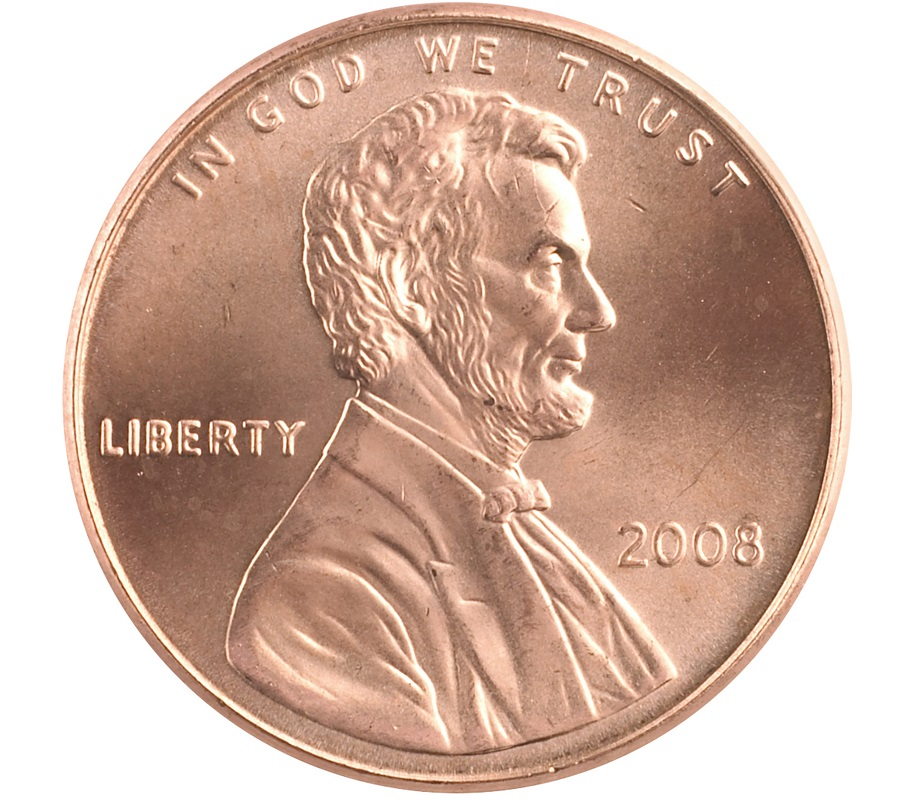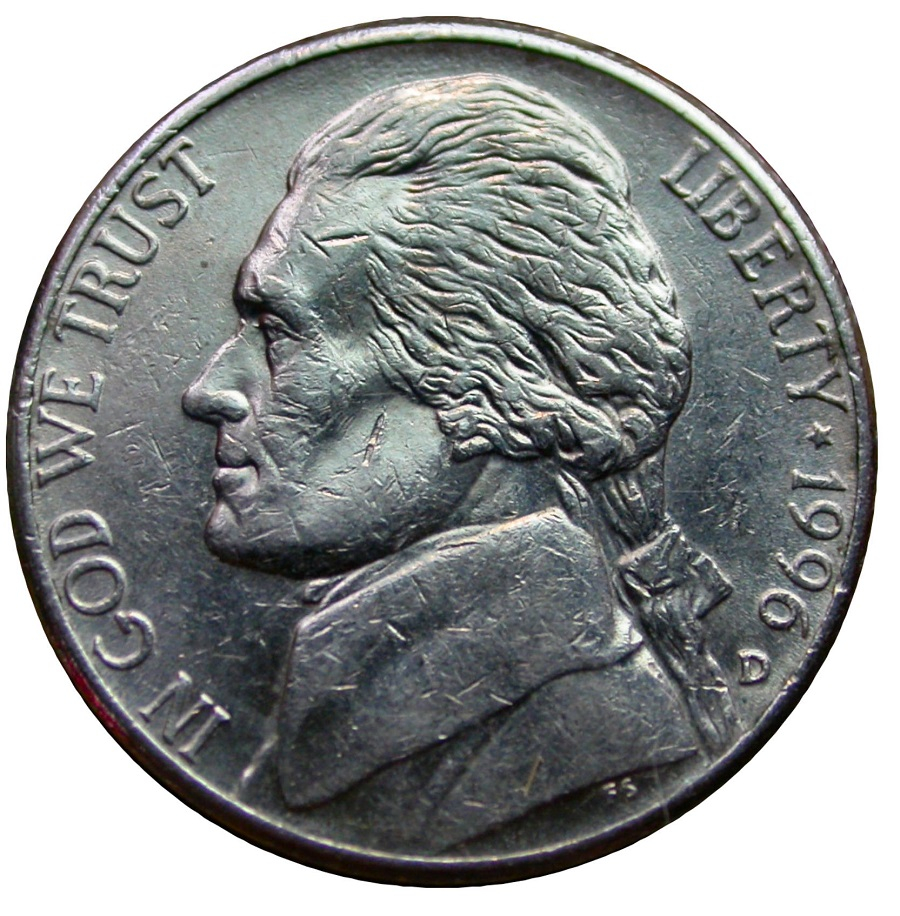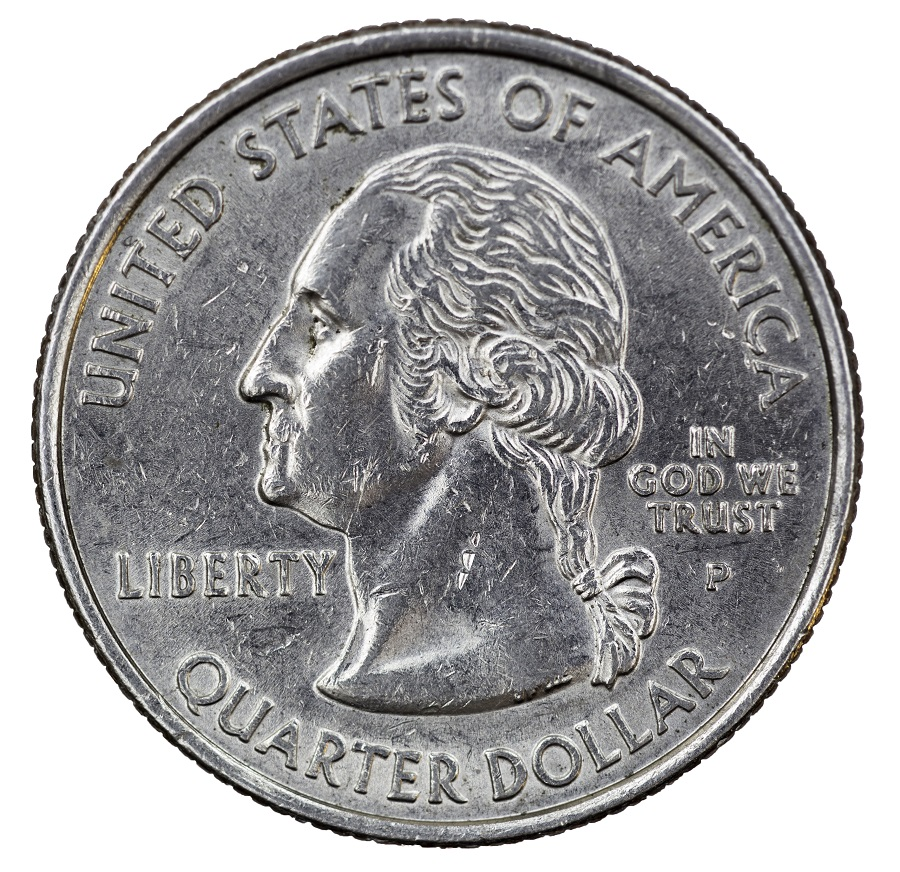# Fraction of a Dollar

Contributor: Erika Wargo. Lesson ID: 12643

Pennies, nickels, dimes, quarters. They are different sizes, and they are different fractions of a dollar. You will learn about fractions and finance with money games and an interactive fraction quiz!

categories

## Fractions and Operations, Measurement and Data

subject
Math
learning style
Visual
personality style
Otter
Primary (K-2), Intermediate (3-5)
Lesson Type
Skill Sharpener

## Lesson Plan - Get It!Jenny has two quarters and Paul has five dimes. Who has more coins? Who has more money?

When writing amounts of money, two forms can be used: cents and dollars.

• To write cents, use a cent sign and do not use a decimal point with it. A quarter is worth 25 cents, or 25¢.
• To write dollars, use a decimal point and dollar sign, \$1.00. A decimal point is also used to show fractions of a dollar. A fraction is part of a whole, so "a fraction of a dollar" is a part of a dollar. A quarter, or \$0.25, means “twenty-five hundredths of a dollar,” which is a fraction of a dollar equal to 25 cents.

Discuss with a parent or teacher the name and value of each coin below:Now that you have discussed the name and value of each coin, let’s look at what “fraction of a dollar” each coin is. Think about how many parts equal a whole. In this case, the parts are the coins and the whole is the dollar:

 Name of Coin Coin Value "Fraction of a Dollar" penny\$0.01 1/100 nickel\$0.05 5/100 dime\$0.10 10/100 quarter\$0.25 25/100

Discuss with a parent or teacher:

• How many pennies equal a dollar?
• How many nickels equal a dollar?
• How many dimes equal a dollar?
• How many quarters equal a dollar?

Now use the table above to work through the examples below:

Example
A penny is what fraction of a dollar?

Since 100 pennies equals one dollar, then one penny is 1/100 of a dollar.

Example
A dime is what fraction of a dollar?

Since ten dimes equals one dollar, then one dime is 1/10 of a dollar.

Example
Three quarters are what fraction of a dollar?

Four quarters equal a dollar, so each quarter is ¼ of a dollar. Three quarters would be ¼ + ¼ + ¼ , or ¾ of a dollar.

Watch this short explanation on Fractions of a Dollar (Quarters):Discuss with a parent or teacher how many quarters would make a dollar.

• How many pennies make up a dollar?
• How do you determine "the fraction of a dollar" that a certain coin is?

Now, you will move on to the Got It? section to complete interactive practice with fractions of a dollar.

## Elephango's Philosophy

We help prepare learners for a future that cannot yet be defined. They must be ready for change, willing to learn and able to think critically. Elephango is designed to create lifelong learners who are ready for that rapidly changing future.